# 1. SIFT (Scale Invariant Feature Transform)

Another scale-invariant algorithm I want to address is the SIFT. With SIFT, the location of local feature points (interest points) are extracted from an image, and further, the corresponding vector representation of the respective interest point is generated, which could be used later on for feature matching between images. As SIFT has quite many steps involved, it might seem complicated and frustrated but many of them are from what we already studied (e.g. feature descriptor like HoG, scale selection for local feature points by LoG).

We will go through the flow of SIFT one by one, but before getting into the detail of each step, I will first give you the bird’s-eye view of SIFT.

(1) Scale-space extrema detection

• Similar to Harris-Laplace detector, SIFT searches interest point candidates over all points in the image and scales, and store a list of computed candidates in form of (x, y, σ). This list will be used for later steps.

(2) Keypoint localization and filtering

• For each interest point candidate, a detailed model is applied to determine the final location and scale of the candidate. Afterward, thresholding is performed to remove unlikely candidates

(3) Orientation assignment

• To ensure the rotation invariance, one or more orientations are assigned to each keypoint using the computed image gradient directions. These assigned orientations are later used to match and align the image patches (regions) around interest points.

(4) Generation of vector representation for interest points

• The local image gradients in the patch (also called window or region) around the key point at the selected scale are measured and transformed into a vector representation using a descriptor.

## Step (1): Scale-space extrema detection

The first step of SIFT algorithm is searching keypoints (x, y, σ) in image over scales. Although the approach of SIFT to find a suitable scale for a point is similar to as Harris-Laplace did, there is a slight modification for cheaper computation.

Step (1.1): Approximation of LoG by Difference of Gaussian (DoG) in automatic scale selection

Recall that in the automatic scale selection where the Laplacian-of-Gaussian (LoG) is chosen as a signature function. A scale σ for a point (x, y) in an image was selected as the suitable scale among other scales, if (x, y, σ) outputs the highest LoG response among 26 points from the same and neighboring scales.

One might remember that the LoG filter can be approximated by the Difference-of-Gaussian (DoG) with fewer computations, meaning that we can efficiently approximate the Laplacian by performing two Gaussian-blurring operations with different variance terms σ and substracting one Gaussian-blurring result by the other one, as is illustrated in the following figure.

In summary, one can consider this process as replacing each layer in the scale space, produced by LoG, with that produced by DoG for the sake of efficient computation.

Step (1.2): Detecting extreme in the scale space generated by Gaussian Pyramid and DoG

Despite the DoG is a decent approximation of LoG, the quality of approximation depends on the choice of two Gaussian variances, σ and 𝒌σ. In order to improve the approximation quality, SIFT conducts more than one DoG operations with increasing Gaussian kernel variances σ.

However, just doing this does not guarantee that SIFT is scale-invariant because Fig 9 is an approximation of one layer from the scale space produced by LoG, meaning that the algorithm currently only looks at the image in one fixed scale. In order to observe the image from many different scales, SIFT integrates the idea of Gaussian Pyramid into this.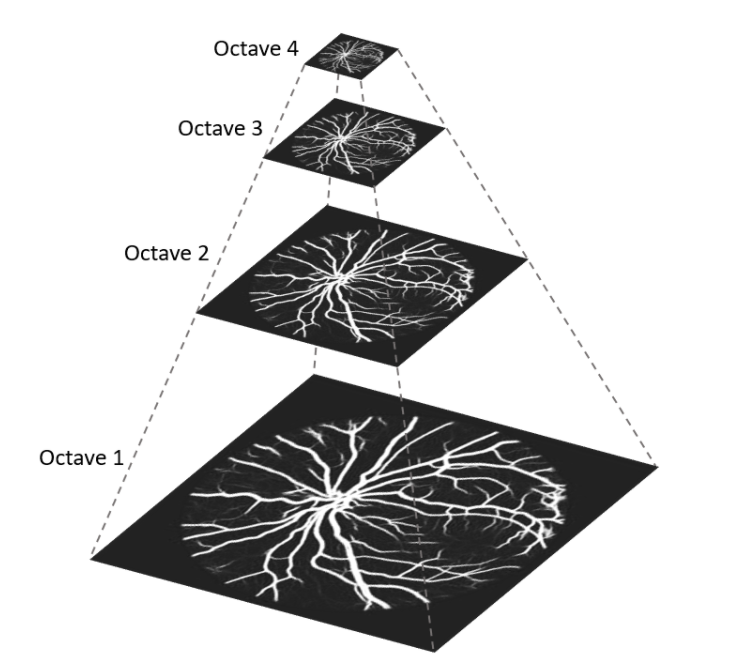Figure 10. Produced Octaves by Gaussian pyramid (sequence of consecutive blurring and sampling), from 

Gaussian pyramid is a multi-scale representation of an image containing a properly re-sized(re-scaled) version of the original image by sampling with Gaussian blurring in order to avoid the aliasing issue. The rescaled results of an image are named as ‘Octave’ in Fig 10.

Given the octaves from Gaussian pyramid, SIFT conducts the operation in Fig 9 with DoG for each octave. As a result, we obtain the complete ‘scale space (see Fig 11)’ which approximates that generated by LoG in Fig 6.

Step (1.3): Local extreme detection

Given the scale space in Fig 11, local extrema (either maxima or minima) are detected by comparing a pixel (red circle) to its 26 neighbors (green) in 3×3 window at the current and adjacent (above and below) scales. More specifically, only a point DoG(x, y, σ) is selected if it is larger or smaller than all of its neighbors. The list of detected extremum (x, y, σ) is the keypoint candidates which will be processed later on in SIFT algorithm.

## Step (2): Keypoint localization and filtering

After finding keypoint candidates by comparing a pixel with its adjacent pixels, the next step is to fit them in a detailed model to more accurately localize the keypoints and filter unlikely keypoints out from the candidate list.

The detailed ‘model’ I referred to is a 3D quadratic scale-space function using the Taylor expansion, which is proposed by Brown and Lowe at 2002, for localization, and 2×2 Hessian matrix for filtering.

Step (2.1): Keypoint localization with Taylor expansion

Let’s mark the detected extrema from step (1) as blue in Fig 13, where the true function and extrema are illustrated. As the scale space produced in step 1 is the discretized version of true-continuous scale space. Therefore, it is not guaranteed that the detected extrema in discrete scale space are the true extrema.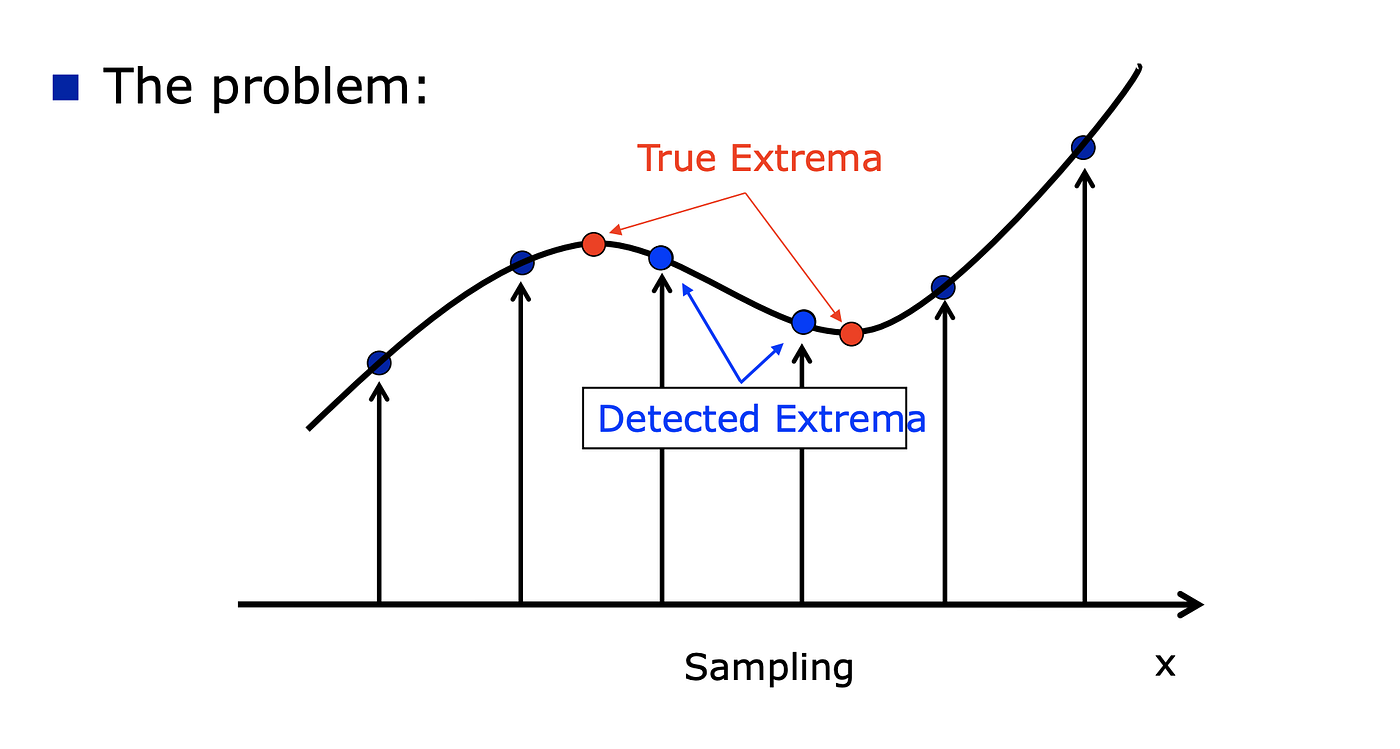Figure 13. Visualization of why the keypoint localization is needed, from 

To solve this and find the location of TRUE extremum, the Taylor expansion is used to get the 3D quadratic approximation of the scale-space function, D(x, y, σ). Afterward, by computing its derivative with respect to x, and finding the point where it goes to zero, give us the location of true extremum. Mathematically, this process can be represented as follows.

Note that, here, the expansion is only up to the quadratic terms.

Step (2.2): Filtering

For stability and computational efficiency, SIFT applies two filtering operations. The first one is removing the detected extrema from 2.1 whose absolute value is less than a certain threshold (in the paper, 0.03). This makes sense because extremum with a low value indicates the extremum has a low contrast to its neighboring points, and therefore, such extremum is unstable and is not important as a local feature.

The second one is eliminating extreme which came from edges. Since the Difference-of-Gaussian, which has a strong response along edges, is used while constructing the scale space, some of the detected extrema are located on an edge even though the edge is poorly determined. Since these extreme makes SIFT instable, the 2×2 Hessian matrix, H, is computed at the location and scale of keypoint (extremum).

Then using the eigenvalues, 𝛂 and 𝜷, of H, it determines whether one eigenvalue is significantly smaller than the other one or not. Recall how the Harris detector detects the edge. If both eigenvalues are large then the point is a corner, and if one is noticeably smaller than the other then it means the point is on an edge.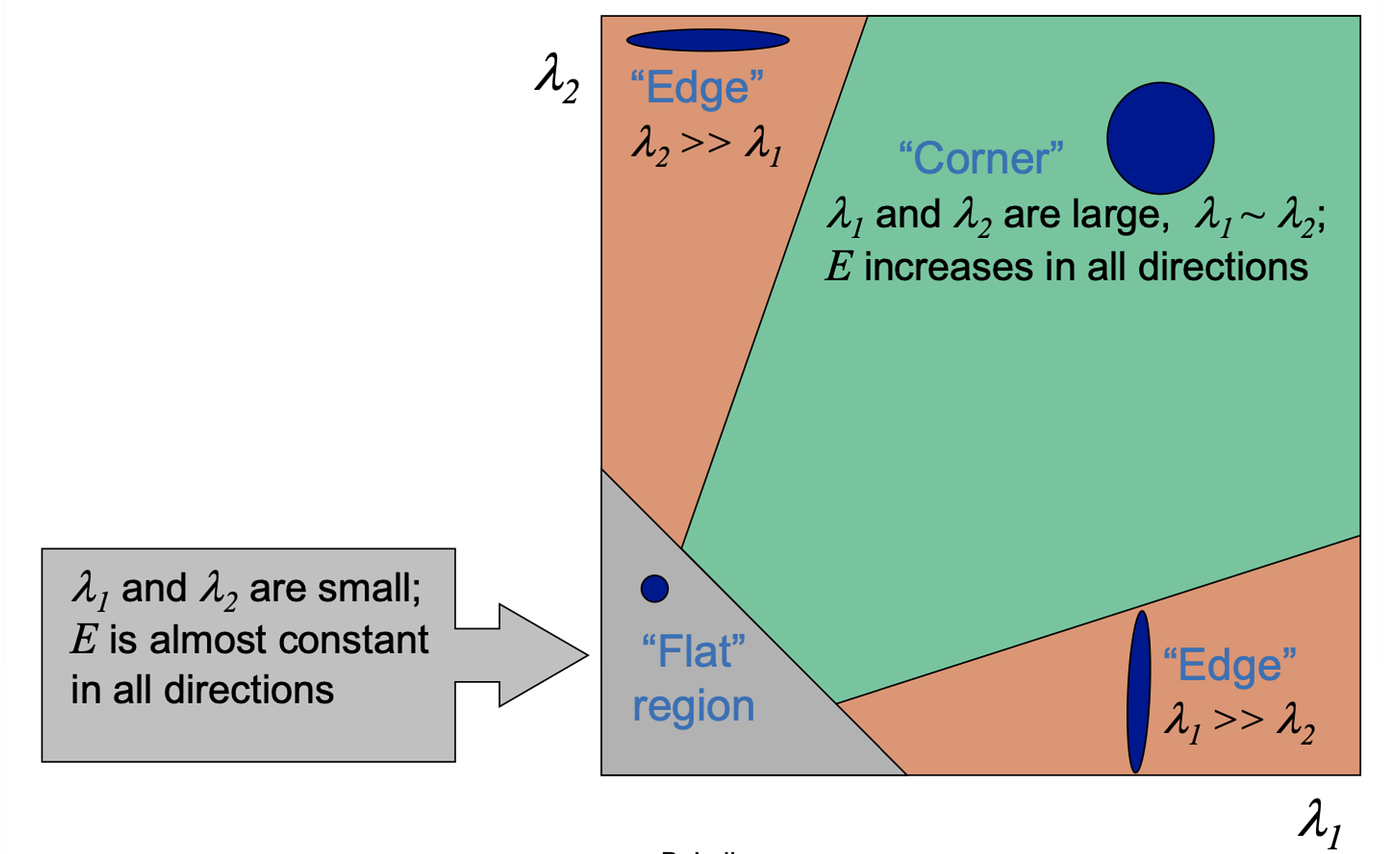Figure 14. Classification of image points using eigenvalues, from [1, 2]

With this idea, SIFT checks all keypoints and tells whether each one of them is a suitable keypoint to be considered as a local feature or not.

After filtering, the number of candidates decreases and only strong and likely keypoints survive. The following figure presents the selected keypoints from each stage of the described steps above.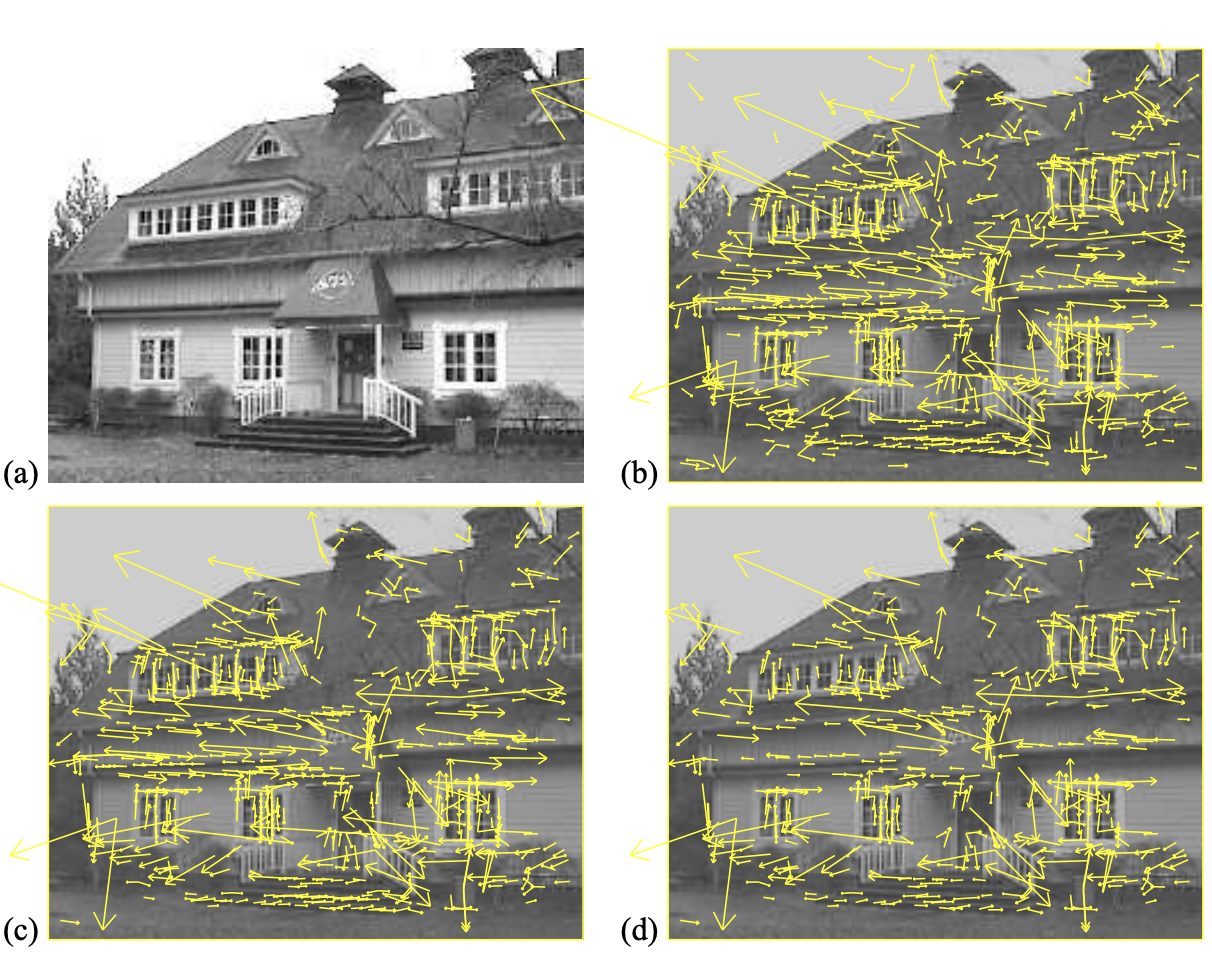Figure 15. keypoint selection. (a) Given image. (b) 832 keypoints location at extreme(maxima and minima) of DoG function in scale space. (c) After removing extreme with low contrast, 729 keypoints survive. (d) After eliminating edge responses, 536 keypoints remain. from 

## Step (3): Orientation assignment

After step (2), a set of good point (x, y, σ) is obtained. σ tells at which Gaussian-blurred image and at which octave in the Gaussian pyramid the extrimum (x, y) is computed. In other words, we can represent the Gaussian-blurred image L from which the extrimum (x, y, σ) is computed as follows:

Then for all sample point in the image L, the gradient magnitude, m, and the orientation, θ, are precomputed using the pixel differences.

Subsquently, 16×16 region (window) around the keypoint is taken, and an orientation histogram is formed. The histogram has 36 bins where each bin covers 10 degrees so that the 360 degree range of orientations are covered. Note that when filling each orientation bin by the gradient magnitude m, the magnitude is weighted by the 2D Gaussian filter whose σ is 1.5 times that of the scale of the keypoint.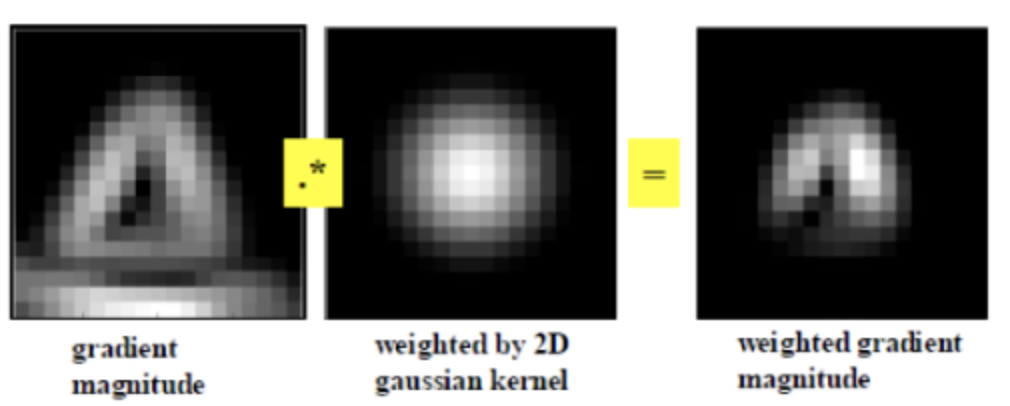Figure 16. Weighted gradient magnitude map by Gaussian kernel which is to be fed into the orientation histogram

Lastly, we need to find the dominant gradient orientation of the region by looking up the highest peak in the histogram. The detected dominant orientation is, then, assigned to the keypoint, and SIFT repeats this orientation assignment process for all keypoints in the set. Therefore, each keypoint has, now, 4 entries: location coordinates, scale, and the assigned orientation (x, y, σ, θ).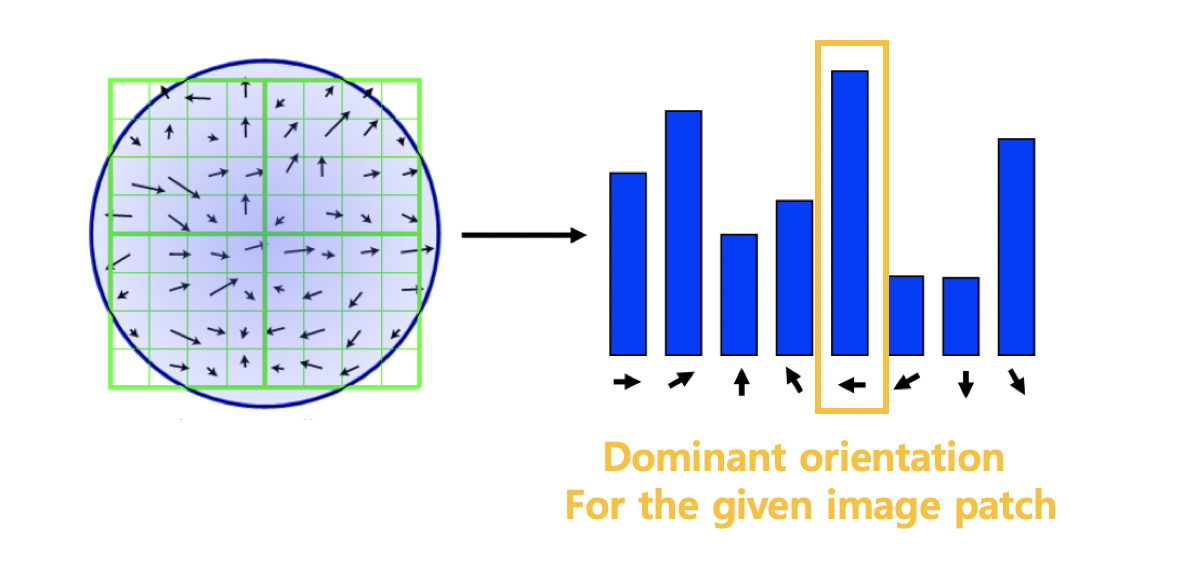Figure 17. Example of detecting the dominant orientation from 8 x 8 region, from 

The orientation assigned to a keypoint will be used in the next step to ensure the rotation invariance of SIFT and when matching keypoints from different images.

## Step (4): Generation of vector representation for interest points

By previous operations, an image location (x, y), scale σ, and orientation θ are assigned to each detected keypoint. As the last step, a local descriptor is computed for a local region around each key point in a similar way to step (3), using the histogram of gradient.

One difference from the histogram of gradient, which is computed in the orientation assignment process in step (3), is that the 16 ×16 region around a keypoint is sub-divided into 16 cells of the size 4×4. The rest parts are the same as step (3). The histogram of gradient is computed for each cell with 8 orientation bins, which are filled by Gaussian-kernel-weighted magnitudes.

Then, a total of 16 histograms from the cells are concatenated to produce the final vector representation of a local keypoint. The generated vector has a length of 16️️*8 (the number of cells * the number of histogram bins).

We are almost there to the end, but there is one operation left. By using Gaussian pyramid and DoG, the local feature descriptors generated by SIFT become scale-invariant. The rotation invariance, however, which is also important, has not yet been achieved.

To ensure the rotation invariance to SIFT, each region around a keypoint (on which the histogram of local gradients is computed) is rotated relative to the assigned keypoint orientation from step (3) so that the dominant orientation points upwards.

By doing this, all local descriptors have the same dominant orientation, and therefore, while computing the similarity between descriptors, SIFT can make an accurate and rotation-invariant comparison.

## This is the end of the flow of SIFT.

In summary, by far we have taken a look at how to extract local features and build descriptors for them in a scale and rotation-invariant manner with Harris-Laplace and SIFT. The computed local descriptor for a keypoint is to be compared with that of another keypoint while feature mapping to determine whether the two keypoints from two different images are the same or not.

It can be done by measuring the similarity between two descriptors, and it gives us the matched keypoint pairs from two images.

One computer vision task, which can be solved by making use of the pairs, is image stitching, meaning how can we properly align or combine two images of the same object, but taken from a different angle, or perspective.

In the next article, I will address how to approach the image stitching using the extract local features.

# Reference

 S. Seitz
 Forsyth & Ponce

 Prof. Svetlana Lazebnik

 Prof M. Irani and R. Basri

 Alexej Efros

 SIFT